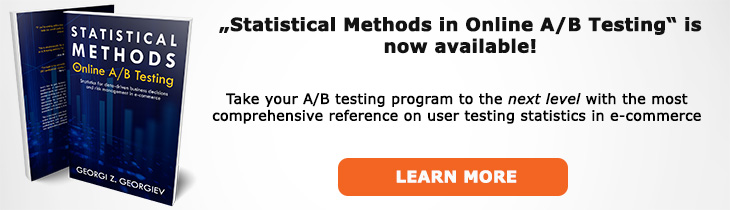# What does "Statistical Power" mean?

Definition of Statistical Power in the context of A/B testing (online controlled experiments).

## What is Statistical Power?

Aliases: sensitivity, power function

The statistical power of an A/B test refers to the test's sensitivity to certain magnitudes of effect sizes. More precisely, it is the probability of observing a statistically significant result at level alpha (α) if a true effect of a certain magnitude (MEI) is in fact present.

When communicated as a single number statistical power is evaluated at a specific alternative hypothesis (magnitude of the difference between the null). However, it is recommended to communicate it by presenting the entire power function over all possible values of the parameter of interest (e.g. all possible absolute or relative differences between the variant(s) and the control).

Statistical power is equal to 1-β where beta is the type II error, or the false negative rate of the test procedure. Thus, power is inversely related to statistical significance while being positively related to the sample size and the minimum effect of interest. Increasing the sample size increases the power against all possible alternatives, increasing the MEI results in a higher power given fixed sample size and significance threshold . Increasing the type I error α results in lower power, assuming the other parameters remain fixed.

Statistical power is also inversely related to the variance and the standard deviation of the metric of interest: higher variance results in lower power, assuming fixed sample size, significance threshold and MEI.

All of these relations become apparent once we consider the full notation of statistical power: POW(T(α); μ1) = P(d(X) > c(α); μ1) for any μ1 greater than μ0, where c(α) is the significance threshold, d(X) is a test statistic (distance function), and μ1 is the magnitude of the effect under a particular alternative hypothesis H1).

## Articles on Statistical Power

Like this glossary entry? For an in-depth and comprehensive reading on A/B testing stats, check out the book "Statistical Methods in Online A/B Testing" by the author of this glossary, Georgi Georgiev.## Glossary Index by Letter

Select a letter to see all A/B testing terms starting with that letter or visit the Glossary homepage to see all.